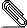# Re: Compiler optimizations questions for ghc 6.10...

```On February 17, 2009 19:24:44 Max Bolingbroke wrote:
> > (compiled with ghc 6.10 with options -O2 -ddump-simpl)```
```
That should have been -ddump-stranal instead of -ddump-simpl.

> > I was wondering why lvl_s1mF is not being inlined into a_s1Gv in the core
> > at the bottom of this email as that is the only place it is ever
> > referenced.
>
> The relevant GHC code is SimplUtils.preInlineUnconditionally. It looks
> like it dosen't get inlined for two reasons:
> 1) It's not a manifest lambda (it's an application) so inlining inside
> another lambda would change the number of times the FVs of lvl_s1mF
> might occur

I have to confess my ignorance here as my google fu failed and so I still
don't know what a manifest lambda is (other than not a application).  : )

> 2) I'm not sure if the use-context is considered interesting by GHC
> because the application of the function might be hidden by the cast.

I was wondering about that, which is why I didn't remove all the cast noise.

> So it looks like the problem stems from digit_s1l3 having arity 1
> rather than arity 3. You could try and force it into a higher arity
> somehow, but I can't say exactly how you might do that without seeing
> the rest of the Core (and in particular the part under the first
> lambda in the definition of digit).

The thing is that the inner lambdas come from inlining that StateT monad
transformers in a StateT q (StateT Int (ErrorT String Identity)) monad (i.e.,
the first one is the q state -- which works out to an Int -- and the second is
the Int state).  I guess I could explicitly pass them around, but that would
seem to defeat the purpose of having StateT.

The actual routines under this implement part of a FSM for (hopefully)
efficiently extracting an Int from a ByteString (or a uvector UArr -- source
of the Step data type).  The relevant part of the actual code, which is a bit
hacked up with ! patterns from my attempts to get better code, is as follows.

type ParseInt q a = StateT q (StateT Int (ErrorT String Identity)) a

next :: q -> Step q Word8
next i | i==n      = Done
| otherwise = Yield (bs `BS.unsafeIndex` i) (i+1)

wrap :: Monad m => (Word8 -> StateT q m a) -> StateT q m a -> StateT q m a
wrap yield (done::StateT q m a) = loop
where loop :: StateT q m a
loop = do q <- get
case next q of
Yield x q' -> put q' >> yield x
Skip    q' -> put q' >> loop
Done       -> done
s2 :: ParseInt q Int
s2 = wrap yield done
where yield :: Word8 -> StateT q (StateT Int (ErrorT String Identity)) Int
yield x | x==48     = digit 0
| x==49     = digit 1
| x==50     = digit 2
| x==51     = digit 3
| x==52     = digit 4
| x==53     = digit 5
| x==54     = digit 6
| x==55     = digit 7
| x==56     = digit 8
| x==57     = digit 9
| otherwise = do !y <- lift get
return y
where digit :: Int -> ParseInt q Int
digit !x = do !y <- lift get
( if y <= (maxBound-9)`quot`10 || y <=
(maxBound-x)`div`10
then let !y' = y*10+x in (lift \$ put y')
>> s2
else throwError "integer overflow" )
done :: ParseInt q Int
done                = do !y <- lift get
return y

I just finished adding the Parse q Int type to help with email line wrapping.
As I alluded to in my original email, if I don't have the Int overflow check
in digit, it is not chosen as the loop breaker, all the StateT stuff is
compiled away, and you get a really nice efficient assembler loop (which is
important because the final FSM has to actually chew through GBs of data).

The part of the code under the first lambda in digit is as follows (I didn't
keep the original dump, so the uniques have changed here).  It's the second
part of the Int overflow bounds check (i.e., y <= (maxBound-x)`div`10), and,
indeed, something you don't want to compute unless the easy check fails.

digit_s1lk =
\ (x_aqR [ALWAYS Just U(L)] :: GHC.Types.Int) ->
case x_aqR
of x_XsQ [ALWAYS Just A] { GHC.Types.I# ipv_s1bD [ALWAYS Just L] ->
let {
lvl_s1my [ALWAYS Just D(T)] :: GHC.Types.Int
[Str: DmdType]
lvl_s1my =
case GHC.Prim.-# 9223372036854775807 ipv_s1bD
of wild2_a1xi [ALWAYS Just L] {
__DEFAULT ->
case GHC.Base.divInt# wild2_a1xi 10
of wild21_a1xj [ALWAYS Just L] { __DEFAULT ->
GHC.Types.I# wild21_a1xj
};
(-9223372036854775808) ->
case GHC.Base.divInt# (-9223372036854775808) 10
of wild21_a1xl [ALWAYS Just L] { __DEFAULT ->
GHC.Types.I# wild21_a1xl
}
} } in
(\ (eta_X1sU [ALWAYS Just L] :: GHC.Types.Int)
(eta_s1G7 [ALWAYS Just U(L)] :: GHC.Types.Int) ->
.
.
.

> In general, the -ddump-inlinings flag is useful for working out why
> something wasn't inlined - but it wouldn't have helped you in this
> case, because it only dumps information about inlining at call sites,
> and you actually want an unconditional inlining to occur.

I also tried that, and didn't have much luck with it.  I didn't understand the
output, which there was 48k lines worth of, and the uniques kept changing
which made it hard to grep for names from previous -ddump-simpl runs.

I'm in the process of reading the "Secrets of the GHC inliner" paper, so,
hopefully, that will at least help me with the first of these problems.  : )

Thanks very much for looking at this and the details reply!

Cheers!  -Tyson
```signature.asc
Description: This is a digitally signed message part.

```_______________________________________________